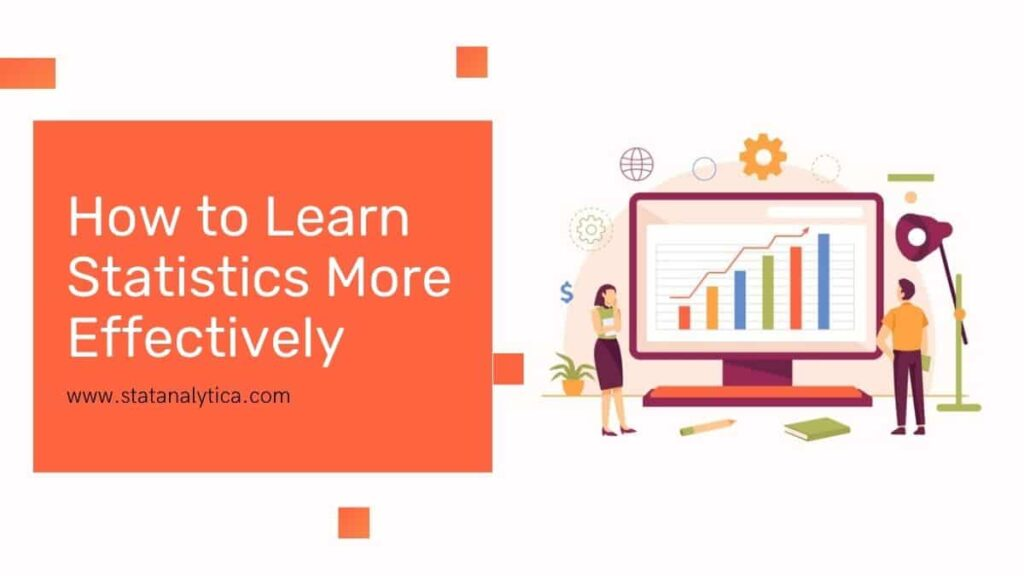# Top 5 Tips on How to Learn Statistics More Effectively

Statistics is a major branch of math. Without statistics, it is impossible to get to the conclusion of the collected data. In fact, statistics is all about data. It helps you to organise the raw data in an efficient manner. Today we will learn how to learn statistics more effectively.

Having a good command over statistics will help you to separate the valuable data from the garbage one. Actually, a non-statistics person may not be able to differentiate between the raw data and the valuable one. All this can only happen with statistics. Let’s dig into the blog and find out how can we learn statistics more effectively.

## Top 5 Tips on How to Learn Statistics More Effectively

### 1. Know the Importance of Statistics

Statistics is an integral part of our daily life. All the industries are using statistics to perform their routine work. For example, in our day to day life, we face statistics likewise when we go for the surgery the doctor tell us what would be the benefits or side effects of the particular surgery.

On the other hand, we daily watch the stats over the internet as well as new channels about the employment rate, GDP, crime rate and so on. There are plenty of examples which we have seen our day to day life.

From this point, you may be able to understand why statistics is crucial for us. And why you need to study statistics. The number of students always have a doubt that why to learn statistics. This point will help them to make sure about “why statistics is important”.

Work on gaining knowledge of statistics: After getting know what is statistics. This is the time to work on gaining the knowledge of statistics.

There are plenty of resources available over the internet and offline that will help you to get a deep knowledge of statistics. You can also gain statistics knowledge with the help of a statistics book.

There are a number of books that will help you to learn statistics from basic to the advanced level.

### 2. Learn the terms most often used in statistical analysis.

#### Mode

It is the set of data values that appear most often in a given dataset. Suppose that if in a data set the x is a discrete random variable, then the mode of x will be the probability mass function that is having the maximum value.

#### Median

It is the simplest measure of central tendency. We arrange the observation from the smallest to the largest value to find the medium of the given data set. In case of the odd number of observations in a given data set then the middle value automatically become the median. In case of even number of observations, the sum of two middle values become the median.

#### Standard Deviation

Standard deviation is all about the measure that is used to quantify the amount of variation of a set of data values.

#### Distribution

Distribution of statistical data sets (or populations) is a list of function that represents all possible values (or intervals) of data and how often it occurs. When the distribution of hierarchical data is conducted, you see the number or percentage of individuals in each group.

#### Bell-shaped curv

An asymmetrical bell-shaped curve that represents the distribution of values, frequencies, or possibilities of a set of data. … Gaussian or general distribution is the usually mathematically well-defined vine curve in statistics and in science.

#### Probability

The probability distribution is a table or an equation that connects each result of a statistical experiment to the probability of an event. Consider a simple experiment in which we flip a coin twice. Suppose random variable X is defined as the result of two coins.

#### Outliers

For example, the farthest point in the above figure is external. A convenient definition of an external is a point that is 1.5 times higher than the interquartile range above or below the first quartile. There can also be out layer when comparing relationships between two sets of data.

### 3. Start applying them to everyday life

The best way to learn anything is from applying it in real life. You can also learn statistics by applying it in your day to day life. You can apply various statistics in your daily life to make solid decisions. In fact, statistics will help you to spend your money more effectively. You can also get various statistics from newspapers, media, politics and sports.

### 4. Learn about statistics from what people telling you and asking them questions

Statistics is one of the toughest subjects for anyone. Whether you are an intelligent student or an average one. When you get into statistics class. Then you can learn statistics more effectively by asking the question from your mentor.

You should also focus on what your mentor is teaching to you. It is the best and most effective way to learn statistics. Your little attention can help you to have a better command over statistics.

### 5. Find some software that will help you manipulate a given set of values

Statistics software are also playing crucial in learning statistics. This software helps you to manipulate data more easily then you do manually.

There are plenty of commands assigned in that software to perform some predefined statistics function. If you have some basic knowledge about statistics then it would help you can operate these statistics tools easily and even learn more about statistics with these tools.

## Conclusion

All the steps mentioned above are quite useful in learning statistics more effectively. If you are a beginner in statistics and want to learn statistics then follow these steps and get a solid command over statistics. You can also take the statistics assignment help that will play a supporting role in learning statistics.